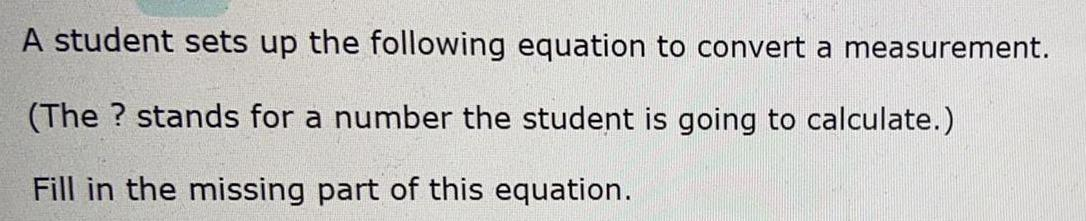Question:

# A student sets up the following equation to convert a

Last updated: 8/4/2022A student sets up the following equation to convert a measurement. (The? stands for a number the student is going to calculate.) Fill in the missing part of this equation.